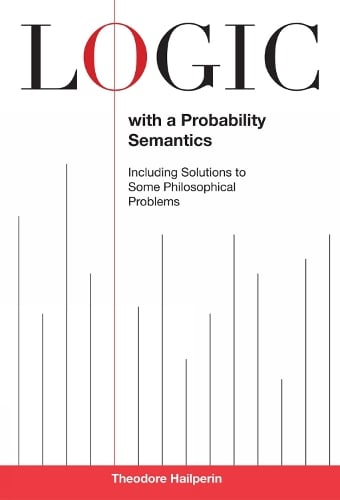•# Logic with a Probability Semantics (Hardback)

(author)
£62.00
Hardback 126 Pages / Published: 16/12/2010
• We can order this from the publisher

UK delivery within 3-4 weeks

The present study is an extension of the topic introduced in Dr. Hailperin's Sentential Probability Logic, where the usual true-false semantics for logic is replaced with one based more on probability, and where values ranging from 0 to 1 are subject to probability axioms. Moreover, as the word "sentential" in the title of that work indicates, the language there under consideration was limited to sentences constructed from atomic (not inner logical components) sentences, by use of sentential connectives ("no," "and," "or," etc.) but not including quantifiers ("for all," "there is"). An initial introduction presents an overview of the book. In chapter one, Halperin presents a summary of results from his earlier book, some of which extends into this work. It also contains a novel treatment of the problem of combining evidence: how does one combine two items of interest for a conclusion-each of which separately impart a probability for the conclusion-so as to have a probability for the conclusion based on taking both of the two items of interest as evidence? Chapter two enlarges the Probability Logic from the first chapter in two respects: the language now includes quantifiers ("for all," and "there is") whose variables range over atomic sentences, not entities as with standard quantifier logic. (Hence its designation: ontological neutral logic.) A set of axioms for this logic is presented. A new sentential notion-the suppositional-in essence due to Thomas Bayes, is adjoined to this logic that later becomes the basis for creating a conditional probability logic. Chapter three opens with a set of four postulates for probability on ontologically neutral quantifier language. Many properties are derived and a fundamental theorem is proved, namely, for any probability model (assignment of probability values to all atomic sentences of the language) there will be a unique extension of the probability values to all closed sentences of the language.

Publisher: Lehigh University Press
ISBN: 9781611460100
Number of pages: 126
Weight: 358 g
Dimensions: 241 x 162 x 15 mm

MEDIA REVIEWS
There are some original features in the treatment given to the subject by the author, which make it an interesting reading also for people well acquainted with other work on probabilistic logics. * Mathematical Reviews *
Anyone interested in the history and philosophy of logic will find this work intriguing. Amateur logicians will find it challenging but will appreciate the progression toward expressing quantified probability logic in a richer formal structure, thus broadening the book's range of possible applications. * Mathematics Teacher *